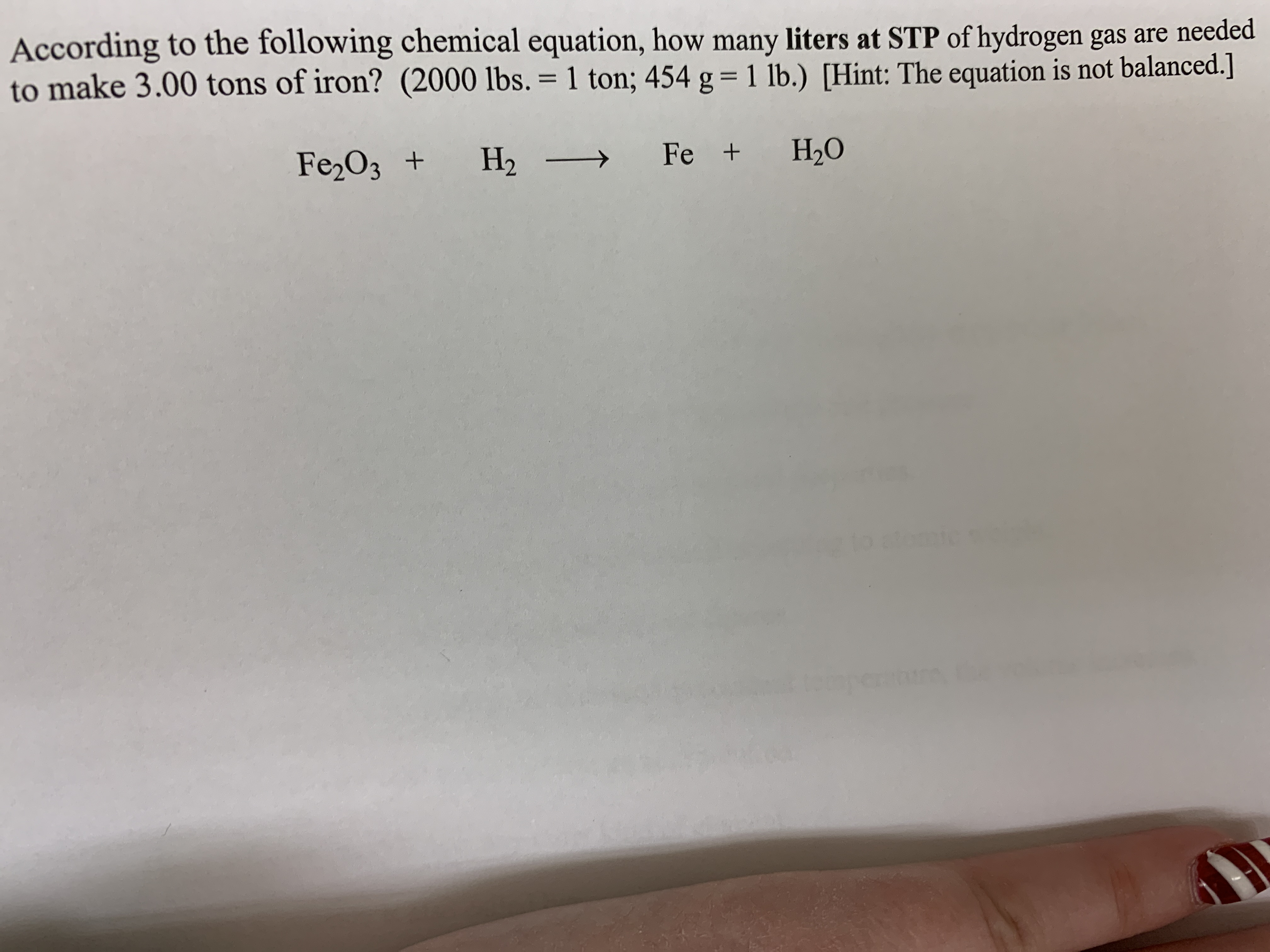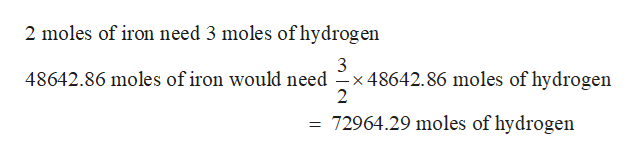# According to the following chemical equation, how many liters at STP of hydrogen gas are neededto make 3.00 tons of iron? (2000 lbs. = 1 ton; 454 g = 1 lb.) [Hint: The equation is not balanced.]%DFe,O3 +Н2Fe +Н.Оomic

Question
2 viewshelp_outlineImage TranscriptioncloseAccording to the following chemical equation, how many liters at STP of hydrogen gas are needed to make 3.00 tons of iron? (2000 lbs. = 1 ton; 454 g = 1 lb.) [Hint: The equation is not balanced.] %D Fe,O3 + Н2 Fe + Н.О omic fullscreen
check_circle

Step 1

Amount of iron to be manufactured = 3 tonnes

The given equation for the reaction is

Step 2

mass of iron given = 3ton x 2000lb/ton x 454g/lb= 2724000g

No of moles of iron to be manufactured

Step 3

Amount of hydrog...help_outlineImage Transcriptionclose2 moles of iron need 3 moles of hydrogen 3 48642.86 moles of iron would need -x 48642.86 moles of hydrogen 2 = 72964.29 moles of hydrogen fullscreen

### Want to see the full answer?

See Solution

#### Want to see this answer and more?

Solutions are written by subject experts who are available 24/7. Questions are typically answered within 1 hour.*

See Solution
*Response times may vary by subject and question.
Tagged in

### Chemistry